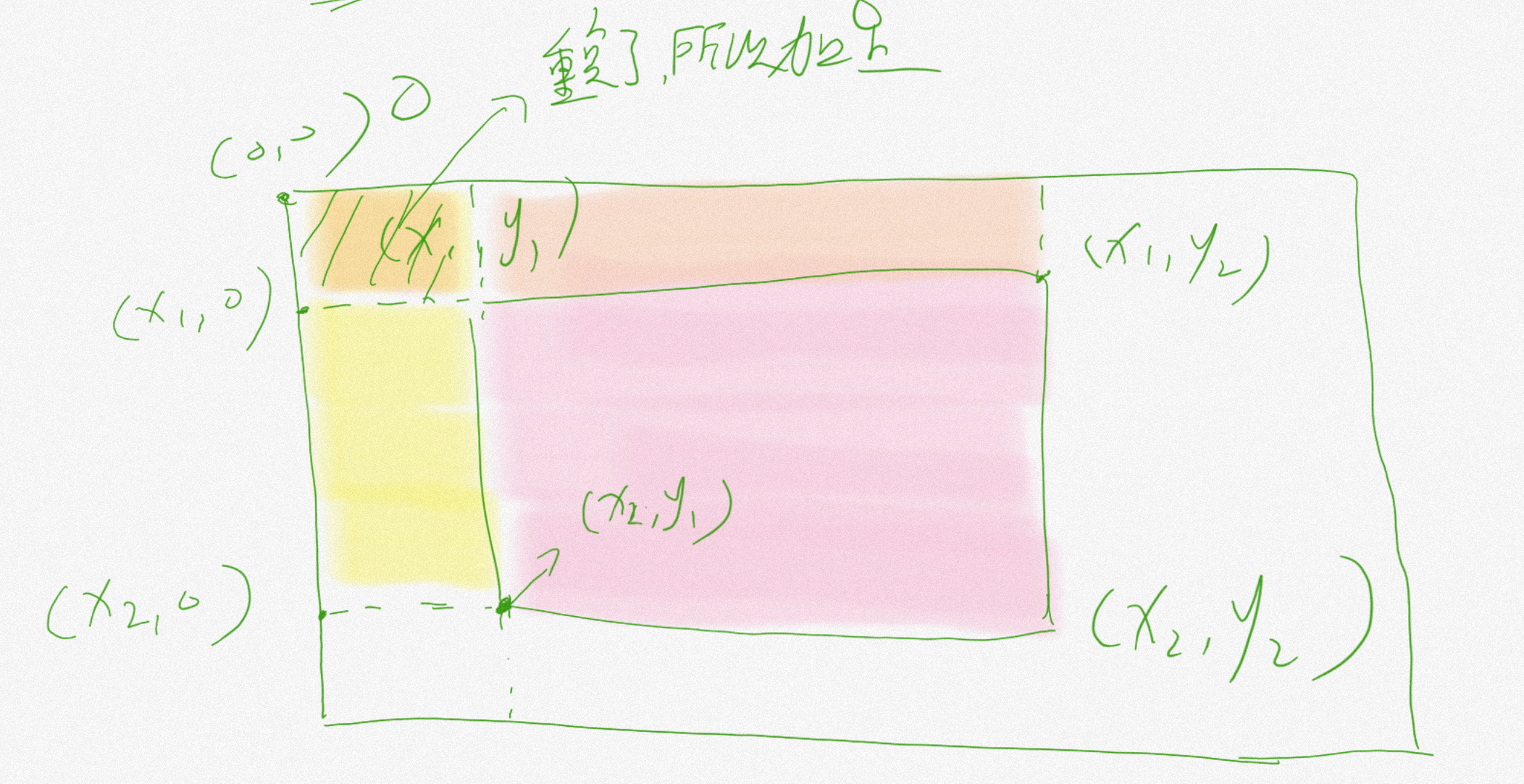# 前缀和与差分¶

## 前言¶

www.bilibili.com/video/BV1xq4y1y7Kz

## 一、前缀和¶

### 1.2一维前缀和¶

#### 1.2.2思路¶

for(int i = 1;i <= n; ++i) {
cin>>a[i];
pre[i] = pre[i-1] + a[i];
}


### 1.3一维前缀积¶

#### 1.3.2思路¶

pre = 1;//否则全都变成0了
for(int i = 1;i <= n; ++i) {
cin>>a[i];
pre[i] = pre[i-1] * a[i] % mod;
}

ans = pre[R] * inv(pre[L-1]);


### 1.5二维前缀和¶

#### 1.5.2思路¶for(int i = 1;i <= n; ++i) {
for(int j = 1;j <= m; ++j) {
cin>>a[i][j];
pre[i][j] = pre[i-1][j] + pre[i][j-1] - pre[i-1][j-1] + a[i][j];
}
}
ans=pre[x2][y2]-pre[x2][y1-1]-pre[x1-1][y2]+pre[x1-1][y1-1];


## 二、差分数组¶

### 2.2性质¶

• 计算数列各项的值：观察$$d=f+f=d+d-d=d$$可知，数列第$$i$$项的值是可以用差分数组的前$$i$$项的和计算的，即$$d[i]=f[i]$$的前缀和。
• $$i$$项的前缀和即为数列前$$i$$项的和